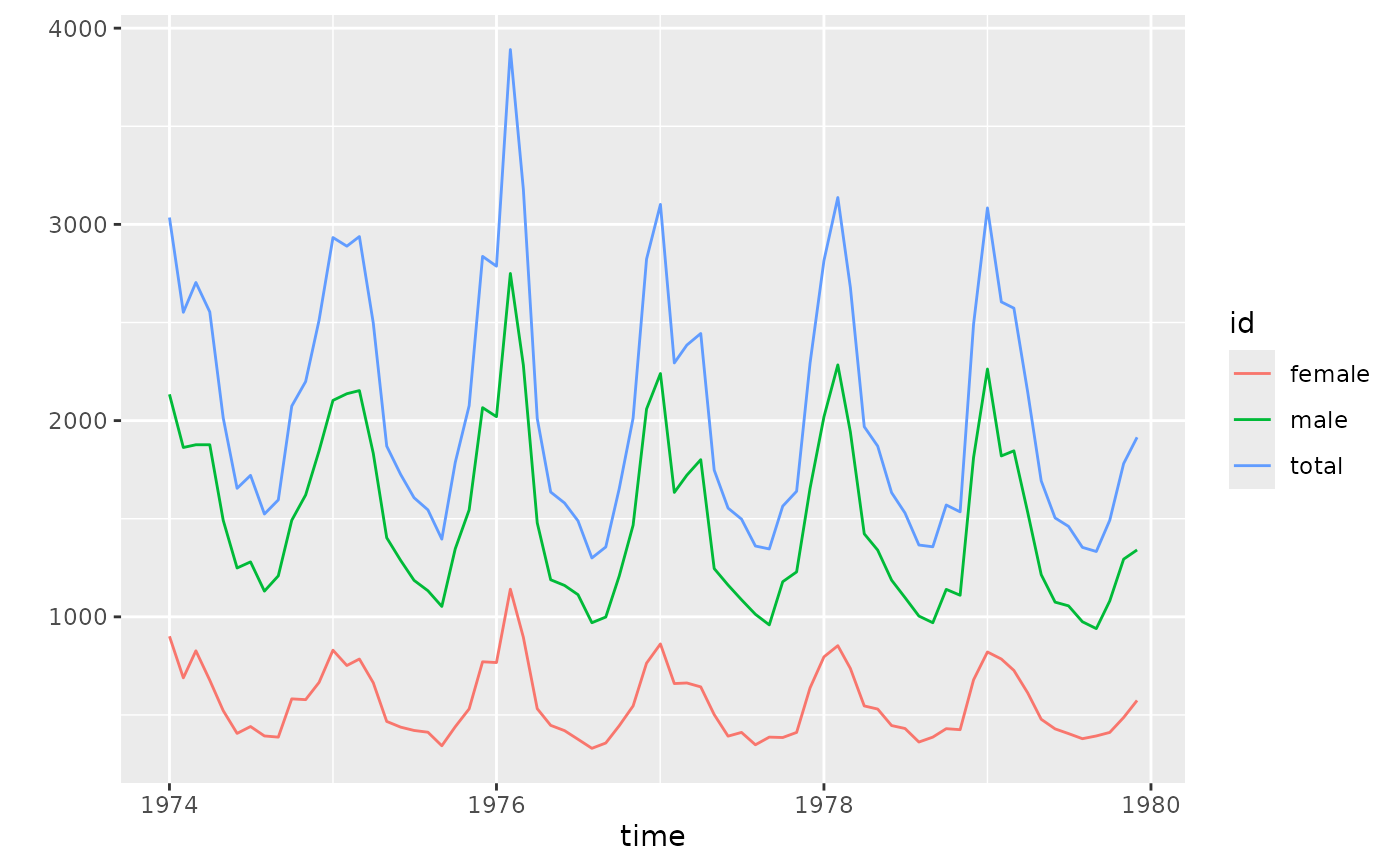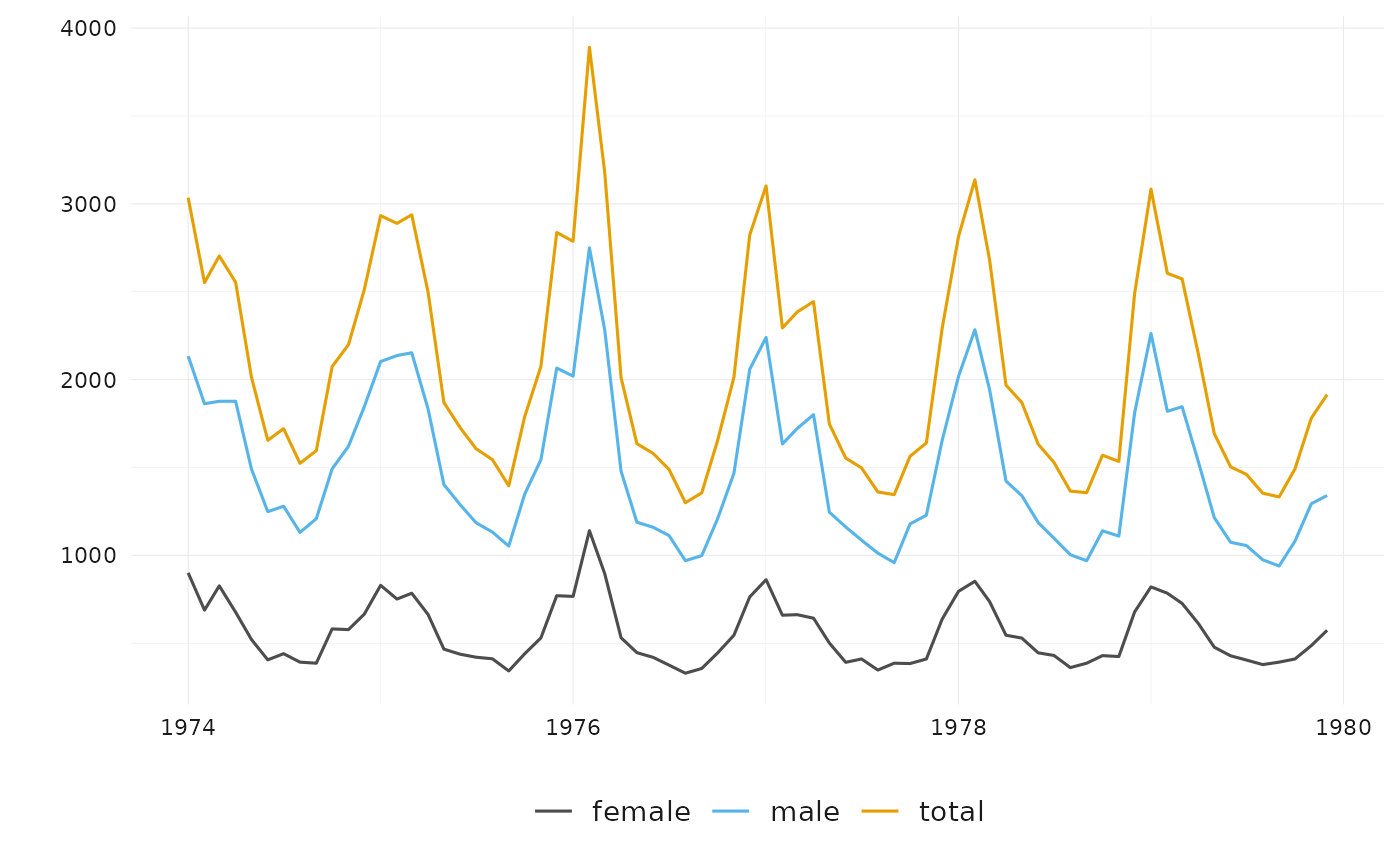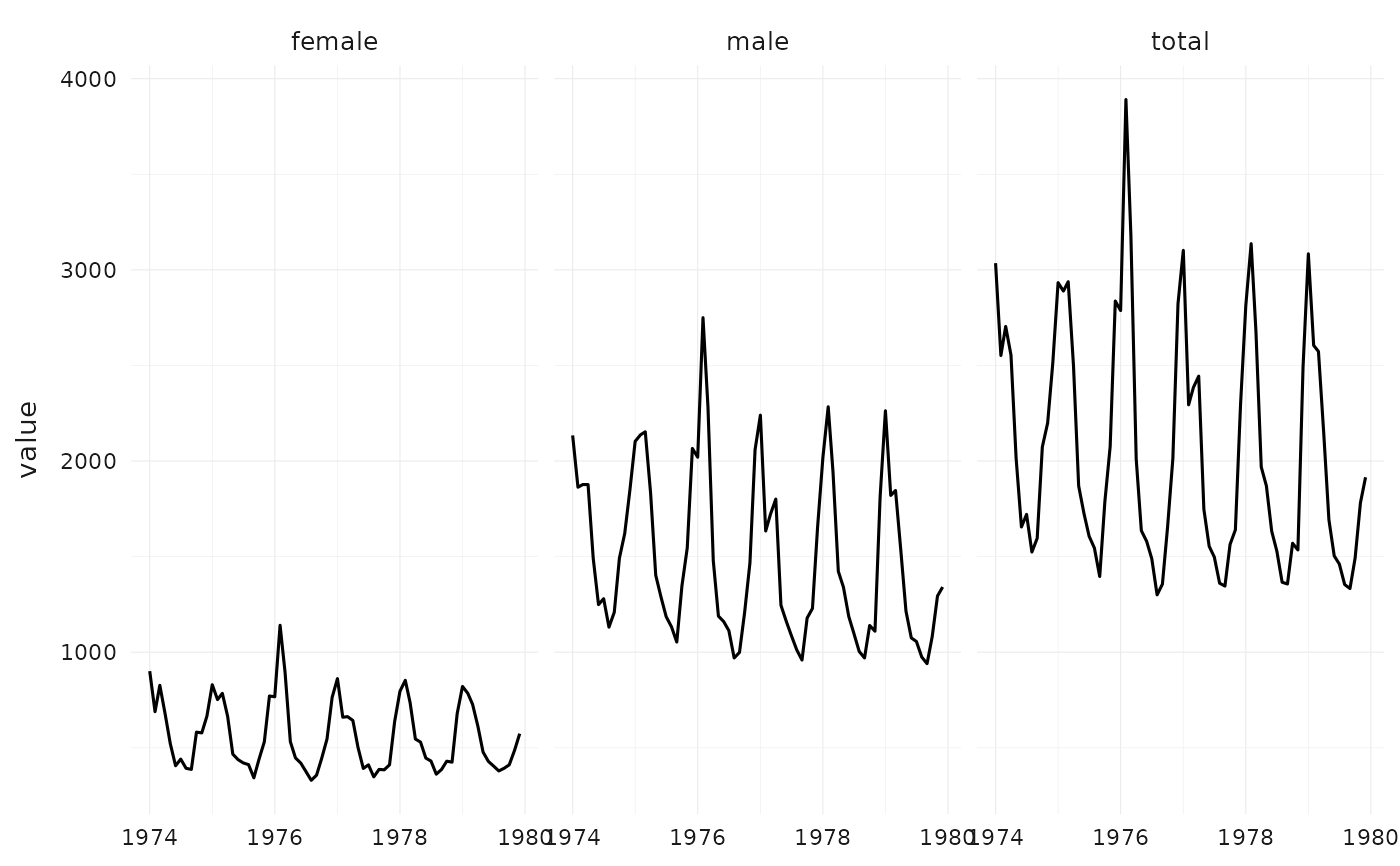`ts_ggplot()` has the same syntax and produces a similar plot as `ts_plot()`, but uses the ggplot2 graphic system, and can be customized. With `theme_tsbox()` and `scale_color_tsbox()`, the output of `ts_ggplot` has a similar look and feel.

## Usage

``````ts_ggplot(..., title, subtitle, ylab = "")

theme_tsbox(base_family = getOption("ts_font", ""), base_size = 12)

colors_tsbox()

scale_color_tsbox(...)

scale_fill_tsbox(...)``````

## Arguments

...

ts-boxable time series, objects of class `ts`, `xts`, `data.frame`, `data.table`, or `tibble`. For `scale_` functions, arguments passed to subfunctions.

title

title (optional)

subtitle

subtitle (optional)

ylab

ylab (optional)

base_family

base font family (can also be set via `options`)

base_size

base font size

## Details

Both `ts_plot()` and `ts_ggplot()` combine multiple ID dimensions into a single dimension. To plot multiple dimensions in different shapes, facets, etc., use standard ggplot (see examples).

`ts_plot()`, for a simpler and faster plotting function. `ts_dygraphs()`, for interactive time series plots.

## Examples

``````# \donttest{
# using the ggplot2 graphic system
p <- ts_ggplot(total = ldeaths, female = fdeaths, male = mdeaths)
p# with themes for the look and feel of ts_plot()
p + theme_tsbox() + scale_color_tsbox()# also use themes with standard ggplot
suppressMessages(library(ggplot2))
df <- ts_df(ts_c(total = ldeaths, female = fdeaths, male = mdeaths))
ggplot(df, aes(x = time, y = value)) +
facet_wrap("id") +
geom_line() +
theme_tsbox() +
scale_color_tsbox()# }

if (FALSE) {
library(dataseries)
dta <- ds(c("GDP.PBRTT.A.R", "CCI.CCIIR"), "xts")
ts_ggplot(ts_scale(ts_span(
ts_c(
`GDP Growth` = ts_pc(dta[, "GDP.PBRTT.A.R"]),
`Consumer Sentiment Index` = dta[, "CCI.CCIIR"]
),
start = "1995-01-01"
))) +
ggplot2::ggtitle("GDP and Consumer Sentiment", subtitle = "normalized") +
theme_tsbox() +
scale_color_tsbox()
}
``````